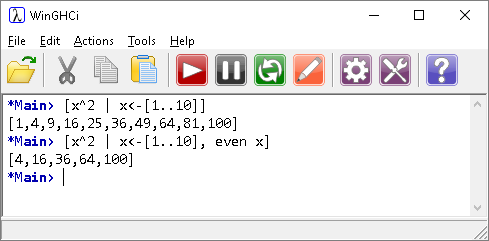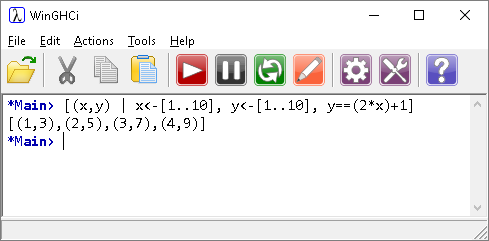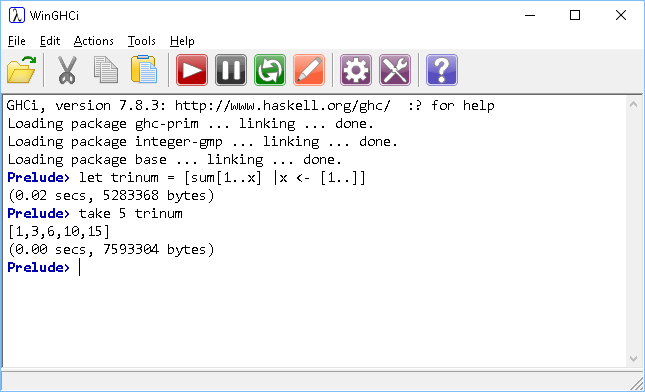# Introduction To Haskell List Comprehensions

## Introduction

List comprehensions are a useful way of creating lists in Haskell.The list comprehension starts with a definition of the output of the list. The pipe separates this from the qualifier for our list comprehension. In this case, we are saying that the local variable x is drawn from the list of numbers 1 to 10.

We can adapt this further by adding a predicate (Boolean expression) to the end of the list comprehension. This is separated from the previous by a comma.The predicate we have added means that our values of x are the even numbers drawn from the list.

You can have more than one predicate and can draw numbers from more than one list.This list comprehension gives a list of co-ordinate pairs that satisfy the equation y = 2x + 1. The co-ordinate pairs in this example are a tuple. Using square brackets instead of curved and you get a list of lists.

Here is an infinite list of triangular numbers, defined using a list comprehension.

```trinum = [sum[1..x] | x <- [1..]]
```